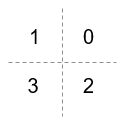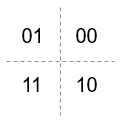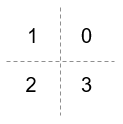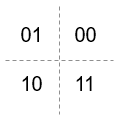# comm.QPSKModulator

Modulate using QPSK method

## Description

The `comm.QPSKModulator` object modulates using the quadrature phase shift keying method. The output is a baseband representation of the modulated signal.

To apply QPSK modulation:

1. Create the `comm.QPSKModulator` object and set its properties.

2. Call the object with arguments, as if it were a function.

## Creation

### Syntax

``qpskmod = comm.QPSKModulator``
``qpskmod = comm.QPSKModulator(Name,Value)``
``qpskmod = comm.QPSKModulator(phase,Name,Value)``

### Description

example

````qpskmod = comm.QPSKModulator` creates a modulator System object™. Use this object to modulate the input signal using the quadrature phase shift keying (QPSK) method.```

example

````qpskmod = comm.QPSKModulator(Name,Value)` creates a QPSK modulator object, with each specified property set to the specified value. You can specify additional name-value pair arguments in any order as (`Name1`,`Value1`,...,`NameN`,`ValueN`).```
````qpskmod = comm.QPSKModulator(phase,Name,Value)` creates a QPSK modulator object, `qpskmod`. This object has the `PhaseOffset` property set to `phase` and the other specified properties set to the specified values. Specify `phase` in radians.```

## Properties

expand all

Unless otherwise indicated, properties are nontunable, which means you cannot change their values after calling the object. Objects lock when you call them, and the `release` function unlocks them.

If a property is tunable, you can change its value at any time.

Phase of zeroth point of the signal constellation in radians, specified as a scalar.

Example: `'PhaseOffset',0` aligns the QPSK signal constellation points on the axes, {(1,0), (0,j), (-1,0), (0,-j)}.

Data Types: `double`

Option to provide input in bits, specified as `false` or `true`.

• When this property is set to `false`, the input values must be integer representations of two-bit input segments and range from 0 to 3.

• When this property is set to `true`, the input must be a binary vector of even length. Element pairs are binary representations of integers.

Data Types: `logical`

Constellation encoding

Signal constellation bit mapping, specified as `'Gray'` or `'Binary'`.

SettingConstellation Mapping for IntegersConstellation Mapping for BitsComment

`Gray`The signal constellation mapping is Gray-encoded.

`Binary`The signal constellation mapping for the input integer `m` (0 ≤ `m` ≤ 3) is the complex value ```e(j*(PhaseOffset) + j*2*π*m/4)```.

Data Types: `char`

Data type assigned to output, specified as `'double'`, `'single'`, or `'Custom'`.

Data Types: `char`

Fixed-Point Properties

Fixed-point data type of output, specified as a `numerictype` (Fixed-Point Designer) object with a signedness of Auto. This property applies when you set the `OutputDataType` property to `Custom`.

#### Dependencies

This property applies when you set the `OutputDataType` property to `'Custom'`.

## Usage

### Syntax

``waveform = qpskmod(insignal)``

### Description

example

````waveform = qpskmod(insignal)` returns baseband-modulated output.```

### Input Arguments

expand all

Input signal, specified as an NS-element column vector of integers or bits, where NS is the number of samples.

The setting of the BitInput property determines the interpretation of the input vector.

Data Types: `double` | `int8` | `logical` | `fi`

### Output Arguments

expand all

Output waveform, returned as a complex-valued vector.

## Object Functions

To use an object function, specify the System object as the first input argument. For example, to release system resources of a System object named `obj`, use this syntax:

`release(obj)`

expand all

 `constellation` Calculate or plot ideal signal constellation
 `step` Run System object algorithm `release` Release resources and allow changes to System object property values and input characteristics `reset` Reset internal states of System object

## Examples

collapse all

Create a QPSK modulator.

`mod = comm.QPSKModulator;`

Determine the reference constellation points.

`refC = constellation(mod)`
```refC = 4×1 complex 0.7071 + 0.7071i -0.7071 + 0.7071i -0.7071 - 0.7071i 0.7071 - 0.7071i ```

Plot the constellation.

`constellation(mod)`Create a PSK demodulator having 0 phase offset.

`demod = comm.QPSKDemodulator('PhaseOffset',0);`

Plot its reference constellation. The `constellation` method works for both modulator and demodulator objects.

`constellation(demod)`Create a QPSK modulator object and a phase noise object.

```qpskModulator = comm.QPSKModulator; phNoise = comm.PhaseNoise('Level',-55,'FrequencyOffset',20,'SampleRate',1000);```

Generate random QPSK data. Pass the signal through the phase noise object.

```d = randi([0 3],1000,1); x = qpskModulator(d); y = phNoise(x);```

Display the constellation diagram of the QPSK signal. The phase noise has introduced a rotational distortion on the constellation diagram.

```constDiagram = comm.ConstellationDiagram; constDiagram(y)```Create QPSK modulator object setting the `BitInput` property to `true`. Display the properties.

`qpskmod = comm.QPSKModulator('BitInput',true)`
```qpskmod = comm.QPSKModulator with properties: PhaseOffset: 0.7854 BitInput: true SymbolMapping: 'Gray' OutputDataType: 'double' ```

Determine the reference constellation points.

`refC = constellation(qpskmod)`
```refC = 4×1 complex 0.7071 + 0.7071i -0.7071 + 0.7071i -0.7071 - 0.7071i 0.7071 - 0.7071i ```

Plot the constellation. Since `BitInput` is `true`, the constellation symbols are label with bit values.

`constellation(qpskmod)`Create QPSK modulator object with default properties settings. Display the properties.

`qpskmod2 = comm.QPSKModulator`
```qpskmod2 = comm.QPSKModulator with properties: PhaseOffset: 0.7854 BitInput: false SymbolMapping: 'Gray' OutputDataType: 'double' ```

Plot constellation with default settings. Since `BitInput` is `false`, the constellation symbols are label with integer values.

`constellation(qpskmod2)`## Extended Capabilities

### Blocks

Introduced in R2012a